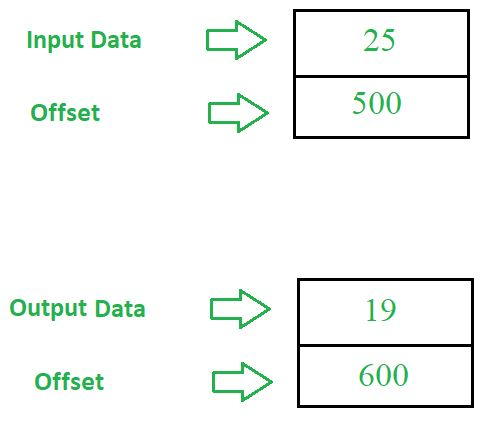Open In App

# 8086 program to convert an 8 bit BCD number into hexadecimal number

Problem – Write an assembly language program in 8086 microprocessor to convert an 8 bit BCD number into hexadecimal number.

Example –Assumption –
Initial value of segment register is 00000.
Memory Address = Segment Register * 10(H) + Offset
where Segment Register is 00000 (Assumption) and Offset is given in the program.

Algorithm –

1. Assign value 500 in SI and 600 in DI.
2. Move the contents of [SI] in BL.
3. Use AND instruction to calculate AND between 0F and contents of BL.
4. Move the contents of [SI] in AL.
5. Use AND instruction to calculate AND between F0 and contents of AL.
6. Move 04 in CL.
7. Use ROR instruction on AL.
8. Move 0A in DL.
9. Use MUL instruction to multiply AL with DL.
11. Move the contents of AL in [DI].
12. Halt the program.

Program –

0400MOV SI, 500SI <- 500
0403MOV DI, 600DI <- 600
0406MOV BL, [SI]BL <- [SI]
0408AND BL, 0FBL = BL AND 0F
040AMOV AL, [SI]AL <- [SI]
040CAND AL, F0BL = AL AND F0
040EMOV CL, 04CL = 04
0410ROR AL, CLRotate AL
0414MUL DLAX = AL * DL
0416ADD AL, BLAL = AL + BL
0418MOV [DI], AL[DI] <- AL
041AHLTEnd of Program

Explanation – Registers used SI, DI, AL, BL, CL, DL.

1. MOV SI,500 is used to move offset 500 to Starting Index(SI)
2. MOV DI,600 is used to move offset 600 to Destination Index(DI)
3. MOV BL,[SI] is used to move the contents of [SI] to BL
4. AND BL,0F is used to mask the higher order nibble from BL
5. MOV AL,[SI] is used to move the contents of [SI] to AL
6. AND AL,F0 is used to mask the lower order nibble from BL
7. MOV CL,04 is used to move 04 to CL
8. ROR AL,CL is used to reverse the contents of AL
9. MOV DL,0A is used to move 0A to DL
10. MUL DL is used to multiply contents of AL with DL
11. ADD AL,BL is used to add contents of AL and BL
12. MOV [DI],AL is used to move the contents of AL to [DI]
13. HLT stops executing the program and halts any further execution